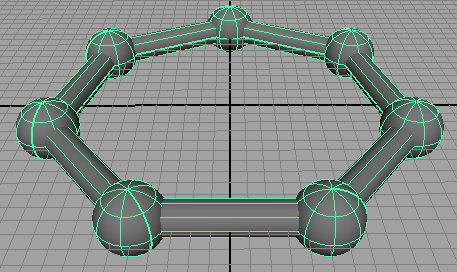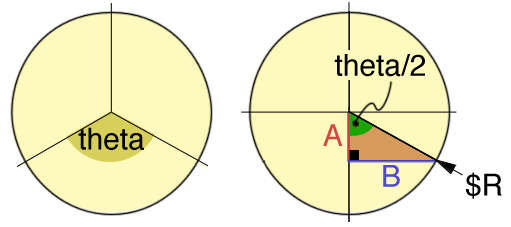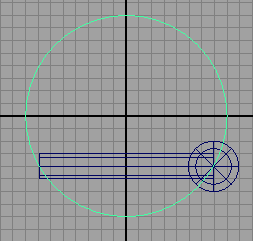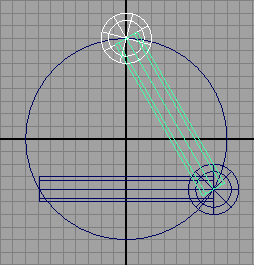### MelModeling with Smart Transforms

#### Introduction

The mel script in listing 1 produces a ring-like structure built from a repetition of spheres and cylinders - figure 1. This tutorial demonstrates how repetitive modeling can be accomplished using instancing and smart transforms.Figure 1

#### Basic Code

Listing 1 provides the code for a procedure that can model a ring consisting of cylinders and spheres. Given the number of radius of the ring (`\$R`) and the number of sides (`\$nsides`) the procedure calculates the angle between the cylinders and their lengths.

Listing 1 (ring.mel)

 ```global proc string ring(int \$nsides, float \$R) { // Give the number of sides and the radius of the "ring" // calculate its proportions float \$theta = 360.0/\$nsides; float \$rads = deg_to_rad(\$theta/2); float \$A = \$R * cos(\$rads); float \$B = \$R * sin(\$rads); float \$ratio = (2 * \$B)/0.5; // cylinder height ratio // The "ring consists of repeated cylinders and spheres string \$c[] = `cylinder -p 0 0 \$A -r .5 -ax 1 0 0 -hr \$ratio`; string \$s[] = `sphere -p \$B 0 \$A -ax 0 1 0`; select \$s \$c; // Use either duplication or instancing //string \$d[] = `duplicate -rr`; string \$d[] = `instance`; rotate -r 0 \$theta 0; // Begin making a array (ie. list) of the items that will // form the "ring" group string \$names[] = { \$s, \$c }; appendStringArray(\$names, \$d, size(\$d)); int \$n = 2; for(\$i = 2; \$i < \$nsides; \$i++) { // Use either duplication or instancing //\$d[] = `duplicate -rr -st`; \$d = `instance -st`; appendStringArray(\$names, \$d, size(\$d)); } // Handy for printing the contents of the group //for(\$i = 0; \$i < size(\$names); \$i++) // print(\$names[\$i] + "\n"); return `group \$names`; }```

#### Calculating the Sides

The calculation of the angle and the length of each cylinder proceeds as follows.Figures 2 & 3

For the purpose of explaining how the angle and length of each cylinder in the "ring" is calculated assume the perimeter of the circle in figure 2 is to be divided into three segments. The angle `theta` can be found by dividing 360 degrees by the number of sides. Since sides `A` and `B` form a right-angled triangle, with the radius of the circle (`\$R`) as the hypotenuse, their lengths can be found using trigonometry.

Irrespective of the size of a right-angled triangle, dividing side `A` by the hypotenuse (`\$R`) yields a ratio that is constant for the included angle (`theta/2`) ie. the sine of the angle.

`    A/\$R = sin(theta/2);`

Since theta is known, side A can be calculated as,

`    A = \$R * sin(theta/2);`

Because the trigonometry procedures in mel measure angles in radians, not degrees, the angle, theta/2, is converted to radians prior to being used by `sin`() and `cos`().Figure 3Figure 4

 Figure 3 shows the result of executing lines 1 to 14 of the procedure `ring`(). The remaining code simply "clones" the original sphere and cylinder using instance() followed by a transformation that rotates the "clone" into its first position. The smart transform flag `-st` ensures the "clones" use the same transformations as the master object from which they were instanced. The `ring`() procedure concludes by grouping the surfaces and returning the name of the group.